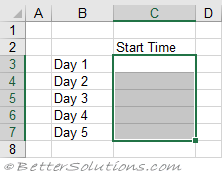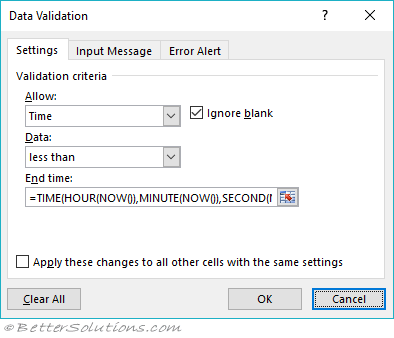# Time Range

### Highlight the cells

This example shows you how to restrict the data entered to time values in a particular range.
Select the cells you want to apply this restruction to. In this case "C3:C7".
In this example we### Enter the Criteria

Press (Data Validation) to display the Data Validation dialog box and select the Settings tab.
Select Time from the Allow drop-down box
Select less than in the data drop-down box.
Enter the following formula for the end time
=TIME(HOUR(NOW()),MINUTE(NOW()),SECOND(NOW()))
The TIME function returns the time as a decimal given the hour, minute and second.
The HOUR function returns the hour as an integer given a date serial number.
The MINUTE function returns the minute as an integer given a date serial number.
The SECOND function returns the number of seconds given a date serial number.
The NOW function returns the serial number of the current system date and time.
This uses the current time as the end time.Press OK to apply the data validation.